• #include "stdio.h" #...//无向图 DispMat( g ); CreateAdj( G, A, nn, e ); printf( "(2)图G的邻接表：\n" ); DispAdj( G ); printf( "深度优先遍历：\n" ); printf( "宽度优先遍历：\n" ); return(1); }
#include "stdio.h"
#include "malloc.h"
#define INF	32767
#define MAXV	100

typedef char InfoType;

typedef struct {
int		no;
InfoType	info;
} VertexType;

typedef struct {
int		edges[MAXV][MAXV];
int		n, e;
VertexType	vexs[MAXV];
} MatGraph;

typedef struct ANode {
struct ANode	* nextarc;
int		weight;
} ArcNode;

typedef struct Vnode {
InfoType	info;
int		count;
ArcNode		* firstarc;
} VNode;

typedef struct {
int	n, e;

/*
* 创建图的邻接矩阵
*/
void CreateMat( MatGraph &g, int A[MAXV][MAXV], int n, int e )
{
int i, j;
g.n	= n;
g.e	= e;
for ( i = 0; i < g.n; i++ )
for ( j = 0; j < g.n; j++ )
g.edges[i][j] = A[i][j];
}

/* 输出邻接矩阵g */
void DispMat( MatGraph g )
{
int i, j;
for ( i = 0; i < g.n; i++ )
{
for ( j = 0; j < g.n; j++ )
if ( g.edges[i][j] != INF )
printf( "%4d", g.edges[i][j] );
else
printf( "%4s", "∞" );
printf( "\n" );
}
}

/*
* 创建图的邻接表
*/
void CreateAdj( AdjGraph * & G, int A[MAXV][MAXV], int n, int e )
{
int	i, j;
ArcNode *p;
for ( i = 0; i < n; i++ )
for ( i = 0; i < n; i++ )
for ( j = n - 1; j >= 0; j-- )
if ( A[i][j] != 0 && A[i][j] != INF )
{
p			= (ArcNode *) malloc( sizeof(ArcNode) );
p->weight		= A[i][j];
}
G->n	= n;
G->e	= e;
}

/* 输出邻接表G */
{
ArcNode *p;
for ( int i = 0; i < G->n; i++ )
{
printf( "%3d->", i );
while ( p != NULL )
{
p = p->nextarc;
}
printf( "∧\n" );
}
}

int main()
{
MatGraph	g;

int nn=0;
printf("input the number of node:\n");
scanf("%d",&nn);
int A[MAXV][MAXV];
printf("input the number of bian:\n");
int bian;
scanf("%d",&bian);
for(int i=0;i<bian;i++){
printf("input the bian: \n");
int o,p;
scanf("%d %d",&o,&p);
A[o][p]=1;
A[p][o]=1;
}
int		n = 6, e = 2;
CreateMat( g, A, nn, e );
printf( "(1)图G的邻接表：\n" );//无向图
DispMat( g );
CreateAdj( G, A, nn, e );
printf( "(2)图G的邻接表：\n" );
printf( "深度优先遍历：\n" );

printf( "宽度优先遍历：\n" );
return(1);
}

展开全文c++ c语言 dfs 算法
• 文章目录带权无向图邻接矩阵表示法(C语言实现)一、邻接矩阵表示法二、本次程序实现的功能三、带权无向图的结构体定义四、创建无向图及邻接矩阵五、输出邻接矩阵六、输出顶点集合七、判断两顶点是否邻接八、全部...
带权无向图的邻接矩阵表示法(C语言实现)

文章目录
带权无向图的邻接矩阵表示法(C语言实现)一、邻接矩阵表示法二、本次程序实现的功能三、带权无向图的结构体定义四、创建无向图及邻接矩阵五、输出邻接矩阵六、输出顶点集合七、判断两顶点是否邻接八、全部代码九、测试

一、邻接矩阵表示法
​ 定义：所谓邻接矩阵存储，是指用一个一维数组存储图中顶点的信息，用一个二维数组存储图中边的信息（即各顶点之间的邻接关系），存储顶点之间邻接关系的二维数组称为邻接矩阵。
​ 对于带权图而言，若顶点Vi 和 Vj 之间有边相连，则邻接矩阵中对应项存放着该边对应的权值，若顶点Vi 和 Vj 不相连，则用0或∞来代表这两个顶点之间不存在边。
​ 例如，对于下面这样一个图：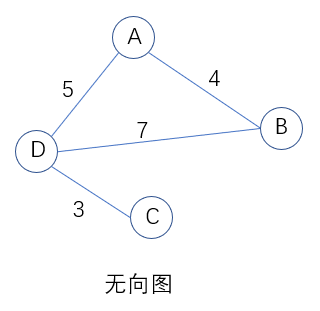​ 我们可以得到其邻接矩阵：
​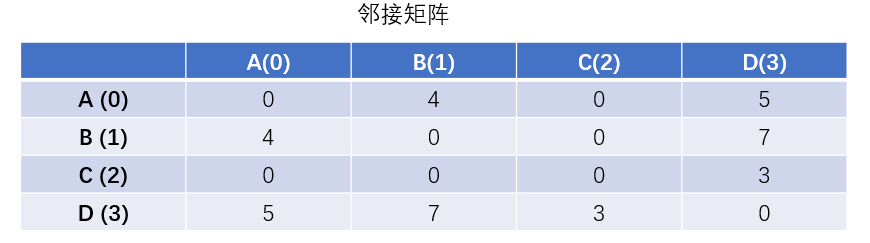​ 注：括号内的0、1、2、3代表其二维数组的下标。
​ 容易发现，带权邻接矩阵有以下特点：①关于主对角线元素对称；②非0的对应位置上的值即为边的权值。
​ 如果是不带权，那么有边的对应位置为1，没边的位置为0，同样也是关于主对角线元素对称。
二、本次程序实现的功能
创建无向图的邻接矩阵   输出无向图对应的邻接矩阵   输出顶点集合   判断两顶点是否邻接，即是否存在直接相连的边
三、带权无向图的结构体定义
typedef char VertexType; //顶点的数据类型
typedef int EdgeType; //带权图中边上权值的数据类型

typedef struct {
VertexType Vex[MaxVertexNum]; //顶点表 MaxVertexNum是最大的顶点数目,下同
EdgeType Edge[MaxVertexNum][MaxVertexNum];//邻接矩阵，边表
int vexnum, arcnum; //图的当前顶点数和边数
}MGraph;//基于邻接矩阵法的带权无向图

四、创建无向图及邻接矩阵
​ 由于比较简单，就不多解释了。值得注意的是，要好好利用无向图的邻接矩阵关于主对角线对称的特性，所以输入边权值时只需要输入上三角或下三角。
void CreateMGraph(MGraph *G)
{
int i,j,k,w;
//先确定顶点数和边数
printf("请输入顶点数和边数,用空格隔开:\n");
scanf("%d %d",&G->vexnum,&G->arcnum);
fflush(stdin);//清空输入缓冲区,否则可能无法正常读取输入

//依次输入顶点的值
printf("请依次输入顶点的值:\n");
for(int i = 0;i < G->vexnum; i++)
{
printf("输入第%d个顶点信息:\n",i+1);
scanf("%c",&G->Vex[i]); //接收值放入顶点表中
fflush(stdin);//清空输入缓冲区,否则可能无法正常读取输入
}

//初始化邻接矩阵
for(i = 0;i < G->vexnum; i++)
for(j = 0;j <G->vexnum; j++)
G->Edge[i][j] = 0;//开始时全部初始化为0,也可以用∞

//建立邻接矩阵
for (k = 0; k < G->arcnum; k++)
{
printf("输入边<vi,vj>的下标i,下标j和权w:\n");
scanf("%d%d%d", &i, &j, &w);	//输入边<vi,vj>上的权值w
G->Edge[i][j] = w;
G->Edge[j][i] = G->Edge[i][j];	//无向图矩阵是对称的
}

}

五、输出邻接矩阵
​ 本质就是遍历一个二维数组。
//输出邻接矩阵
void PrintMatrix(MGraph G)
{
int i,j;
printf("邻接矩阵表示如下:\n");
for (i = 0; i < G.vexnum; i++)
{
for (j = 0; j < G.vexnum; j++)
printf("%-10d", G.Edge[i][j]);//左对齐输出
printf("\n");
}
}

六、输出顶点集合
​ 本质是遍历一维数组。
//输出顶点集合
void PrintVex(MGraph G)
{
printf("\n顶点集合为：");
for(int i=0;i<G.vexnum;i++)
printf("%c ",G.Vex[i]);
printf("\n");
}

七、判断两顶点是否邻接
​ 接收的参数是两个顶点的值，因此需要在顶点表中找到其下标，然后判断其对应位置的邻接矩阵的值是否大于0，如果是大于0即说明邻接，否则不邻接。
​ 注：如果找顶点的下标操作比较频繁，可以考虑再封装成一个函数。
//判断两个顶点之间是否邻接
bool Is_Edge_Exist(MGraph G, VertexType d1, VertexType d2)
{
int i,j,k;
for(k=0;k<G.vexnum;k++)
{
if(G.Vex[k]==d1)
i = k;//找到顶点对应的下标
if(G.Vex[k]==d2)
j = k;//找到顶点对应的下标
}
return G.Edge[i][j]>0?1:0;
}

八、全部代码
#include<stdio.h>
#define MaxVertexNum 10 //顶点数目的最大值
#include<stdbool.h> //根据C99标准,C语言使用bool类型需要添加这个头文件

typedef char VertexType; //顶点的数据类型
typedef int EdgeType; //带权图中边上权值的数据类型

typedef struct {
VertexType Vex[MaxVertexNum]; //顶点表
EdgeType Edge[MaxVertexNum][MaxVertexNum];//邻接矩阵，边表
int vexnum, arcnum; //图的当前顶点数和边数
}MGraph;//基于邻接矩阵法的带权无向图

void CreateMGraph(MGraph *G)
{
int i,j,k,w;
//先确定顶点数和边数
printf("请输入顶点数和边数,用空格隔开:\n");
scanf("%d %d",&G->vexnum,&G->arcnum);
fflush(stdin);//清空输入缓冲区,否则可能无法正常读取输入

//依次输入顶点的值
printf("请依次输入顶点的值:\n");
for(int i = 0;i < G->vexnum; i++)
{
printf("输入第%d个顶点信息:\n",i+1);
scanf("%c",&G->Vex[i]); //接收值放入顶点表中
fflush(stdin);//清空输入缓冲区,否则可能无法正常读取输入
}

//初始化邻接矩阵
for(i = 0;i < G->vexnum; i++)
for(j = 0;j <G->vexnum; j++)
G->Edge[i][j] = 0;//开始时全部初始化为0,也可以用∞

//建立邻接矩阵
for (k = 0; k < G->arcnum; k++)
{
printf("输入边<vi,vj>的下标i,下标j和权w:\n");
scanf("%d%d%d", &i, &j, &w);	//输入边<vi,vj>上的权值w
G->Edge[i][j] = w;
G->Edge[j][i] = G->Edge[i][j];	//无向图矩阵是对称的
}

}

//输出邻接矩阵
void PrintMatrix(MGraph G)
{
int i,j;
printf("邻接矩阵表示如下:\n");
for (i = 0; i < G.vexnum; i++)
{
for (j = 0; j < G.vexnum; j++)
printf("%-10d", G.Edge[i][j]);//左对齐输出
printf("\n");
}
}

//输出顶点集合
void PrintVex(MGraph G)
{
printf("\n顶点集合为：");
for(int i=0;i<G.vexnum;i++)
printf("%c ",G.Vex[i]);
printf("\n");
}

//判断两个顶点之间是否邻接
bool Is_Edge_Exist(MGraph G, VertexType d1, VertexType d2)
{
int i,j,k;
for(k=0;k<G.vexnum;k++)
{
if(G.Vex[k]==d1)
i = k;//找到顶点对应的下标
if(G.Vex[k]==d2)
j = k;//找到顶点对应的下标
}
return G.Edge[i][j]>0?1:0;
}

int main()
{
MGraph G;//无向图
CreateMGraph(&G);//创建图
PrintMatrix(G);//输出邻接矩阵
PrintVex(G);//输出顶点

//判断两个顶点是否邻接
VertexType d1,d2;
d1 = 'A';
d2 = 'B';
if(Is_Edge_Exist(G,d1,d2))
printf("\n%c和%c邻接!\n",d1,d2);
else
printf("\n%c和%c不邻接!\n",d1,d2);
d2 = 'C';
if(Is_Edge_Exist(G,d1,d2))
printf("\n%c和%c邻接!\n",d1,d2);
else
printf("\n%c和%c不邻接!\n",d1,d2);
return 0;
}

九、测试
输入示例：输入时只需要输入上三角部分或下三角部分(不含主对角线上的)即可。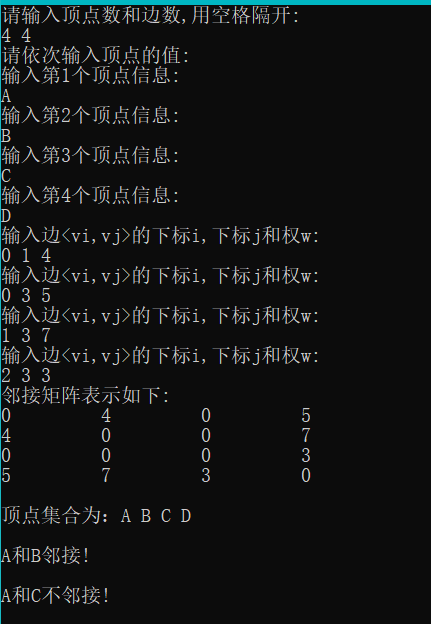展开全文c语言
• 设计并验证如下算法：带权图采用邻接表表示，实现无向图的广度优先搜索与有向图的深度优先搜索。 #define MAX_VERTEX_NUM 20 //图的邻接表存储表示 typedef struct ArcNode{ int adjvex； //该弧所指向的顶点的...
设计并验证如下算法：带权图采用邻接表表示，实现无向图的广度优先搜索与有向图的深度优先搜索。
#define MAX_VERTEX_NUM 20 //图的邻接表存储表示 typedef struct ArcNode{ int adjvex； //该弧所指向的顶点的位置 struct ArcNode *nextarc； //指向下一条弧的指针 InfoType *info； //该弧相关信息的指针 }ArcNode； typedef struct VNode { VertexType data； //顶点信息 ArcNode *firstarc； //指向第一条依附该顶点弧的指针 }VNode，AdjList[MAX_VERTEX_NUM] Typedef struct { AdjList vertices； int vexnum，arcnum； //图的当前顶点数和弧数 int kind； //图的种类标志 }ALGraph；
以上是题目以及题目给的提示。
#include<stdio.h>
#include<stdlib.h>
#define MAX_VERTEX_NUM 20	//图的邻接表存储表示
#define InfoType int
#define VertexType char

typedef struct ArcNode{
struct ArcNode *nextarc; 	//指向下一条弧的指针
InfoType *info; 	//该弧相关信息的指针
}ArcNode;

typedef struct VNode {
VertexType data;	//顶点信息
ArcNode *firstarc; 	//指向第一条依附该顶点弧的指针

typedef struct {
int vexnum,arcnum; 	//图的当前顶点数和弧数
int kind; 	//图的种类标志
}ALGraph;
int vs={0,};

int LocateVex(ALGraph G,char v){//定位函数
for(int i=0;i<G.vexnum;i++){
if(v==G.vertices[i].data)
return i;
}
printf("input error ! input again:\n");
scanf("%c",&v);
getchar();
LocateVex(G,v);
return -1;
}

void PrintUDG(ALGraph G){//输出邻接表
int i,j;
for(i=0;i<G.vexnum;i++){
printf("%d=%c:",i,G.vertices[i].data);
ArcNode *p;
p=G.vertices[i].firstarc;
while(p!=NULL){
p=p->nextarc;
}
printf("\n");
}
}

void create(ALGraph &p)
{
printf("input the graph's kind (1 Undirected 2 Directed):\n");
scanf("%d",&p.kind);
printf("input the graph's vex and arc . \n");
scanf("%d%d",&p.vexnum,&p.arcnum);
getchar();
for(int i=0;i<p.vexnum;i++){
printf("vertex(%d): \n" ,i);
scanf("%c",&p.vertices[i].data);
getchar();
p.vertices[i].firstarc=NULL;
}
char v1 ,v2;
int k ,j;
for(int i=0;i<p.arcnum;i++){
printf("input two vertex :\n");
v1 = getchar();
getchar();
v2 = getchar();
getchar();
j = LocateVex(p, v1);//定位
k = LocateVex(p, v2);
ArcNode *q = (ArcNode *)malloc(sizeof(ArcNode));//申请一个结点
q->nextarc=p.vertices[j].firstarc;//连接结点
p.vertices[j].firstarc=q;//连接结点
if(p.kind==1){//如果是无向图
ArcNode *g = (ArcNode *)malloc(sizeof(ArcNode));//申请一个结点
g->nextarc=p.vertices[k].firstarc;//连接结点
p.vertices[k].firstarc=g;//连接结点
}
}
}

int visit={0};

ArcNode *p;
visit[v]=1;
printf("%d ",v);
p=G[v].firstarc;
while(p!=NULL){
}
p=p->nextarc;//p指向顶点v的下一个邻接点
}
}

{
ArcNode *p;
int Qu,front,rear;//定义循环队列
int visited={0};//使用数组模拟队列
int w;
front=rear=0;//初始化队列
printf("%d ",v);
visited[v]=1;
rear=(rear+1);
Qu[rear]=v;//v进队
while(front!=rear){
front=(front+1);
w=Qu[front];//出队并赋给w
p=G[w].firstarc;//找与顶点w邻接的第一个顶点
while(p){
rear=(rear+1);//该顶点进队
}
p=p->nextarc;
}
}
}


程序运行说明： 1.先输入1或者2构造的图的类型。 2.然后输入顶点个数与弧的个数。 3.再依次输入顶点的名称例如：A B C 4.再输入两个顶点的名称，构造图。 5.最后输入一个起始的点的序号。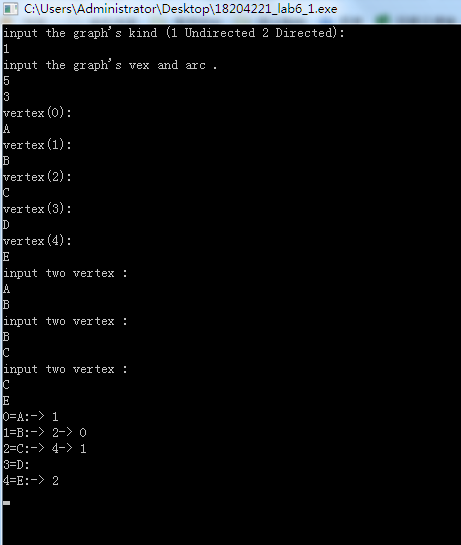最后一个比如你想输入起始点为A点，那你就输入1. 有向图与无向图一样输入 有什么问题欢迎留言，看到就会回答。。
展开全文算法
• 理论上有向图解决了，无向图也可以被解决。 输入格式 4 5 1 4 9 4 3 8 1 2 5 2 4 6 1 3 7 输入时点也可以是点的名称，此时需要建立点的名称字符串与点号的映射。 方法解读 i 号点的第一条边边号为 first[i]；...
有向图需要用十字邻接表来表示。但用链表实现十字邻接表是非常苦恼的，代码很容易便会达到数百行。事实上有一种数组实现十字邻接表，不用指针便可解决有向带权图的问题（含重边，自环）。 理论上有向图解决了，无向图也可以被解决。
输入格式
4 5
1 4 9
4 3 8
1 2 5
2 4 6
1 3 7

输入时点也可以是点的名称，此时需要建立点的名称字符串与点号的映射表。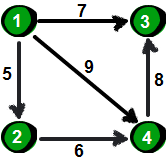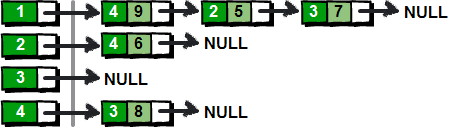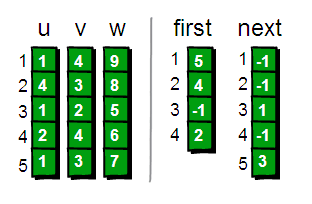方法解读
i 号点的第一条边边号为 first[i]； i 号边的出点的下一条边边号为 next[i]；
特点
① 以边号为主要操作对象。 寻找边的方法是在表中先得到边号，再用u、v、w数组由边号得到边的信息。 ② 反序入表。 各个边在表中的排列顺序与读入顺序恰相反，这是因为每次插入新边都是在链头而非链尾插入。 ③ 放弃数组位置。 为了建立起边号、点号与索引的直接对应关系，建议直接从开始使用。 注： 点号指的是读入时代表点的数字（从多少开始计由输入决定，甚至无需连续。但超大数组大小需能保证容纳所有点号，也可先把点号视作字符串名称记录再建立点号与字符串名称的映射表）； 边号指的是读入时该边是第几个读入的边（从1开始计）。
基本操作实现
0. InitialGraph
//全局变量  ps：我也不想这么野蛮 我也是被带坏的
int u, v, w;	//边的信息
bool flag;						//边是否被访问

//图的初始化：
for (i = 1; i <= M; i++) {
first[i] = -1;
flag[i] = false;
}

1. BuildGraph
有向图：
    for (i = 1; i <= N; i++) {			// i = 1 开始 弃置数组位置
scanf("%d %d %d", &u[i], &v[i], &w[i]);

next[i] = first[u[i]];
first[u[i]] = i;
}

无向图：
    for (i = 1; i <= N; i++) {			// i = 1 开始 弃置数组位置
scanf("%d %d %d",&u[i],&v[i],&w[i]);

next[i] = first[u[i]];
first[u[i]] = i;

//对 i + N 做一遍刚才所做的事  且u v互换(反向)  (N是边数)
v[i + N] = u[i]; u[i + N] = v[i]; w[i + N] = w[i];
next[i + N] = first[u[i + N]];
first[u[i + N]] = i + N;
}

2. 遍历 i 号点的所有边
有向图：
	k = first[i];		// k 初始化为第一条边边号
while (k != -1) {
...
...
k = next[k];	// k 更新为下一条边边号
}

3.遍历全图所有边（对所有点，遍历点的所有边）
for (i = 1; i <= n; i++) {				// i = 1 开始 弃置数组位置
k = first[i];
while (k != -1)
{
...
...
k = next[k];
}
}


（图源https://www.cnblogs.com/codingmengmeng/p/5645073.html）

展开全文数组
•算法 c++
• //AdjList表示邻接表类型 typedef struct//邻接表 { AdjList vertices; int vexnum,arcnum;//的当前顶点数和边数 }ALGraph; void InitGraph(ALGraph &G)//的初始化 { int i; for(i=0;i;i++) G.vertices[i]...c语言链表 算法
• 建立一个带权无向图邻接矩阵表示，判断此图是否连通，若是连通图，用Prim算法输出该图的最小生成树
• @【数据结构】（带权无向图+最小生成树） 带权值的无向图，实现输入和输出，并求出该图的最小生成树。 #include<iostream> #include<stdio.h> #include<stdlib.h> #include<math.h> #...算法
• c语言实现数据结构中有向图和无向图邻接表的建立、插入、删除操作c语言 算法 指针
• 图邻接表结构： 邻接表用链表来存储邻接点（分两个域，一个存顶点下标，一个存下一个邻接点的引用），通过一个类（我用了内部类，所以是private）定义邻接点： private class AdgvexType { int verNum = -1...java
• Prim算法计算最小生成树（无向图&邻接矩阵）——C语言实现。
• 比如有个这样的无向图（看起来很像二叉树吧，其实二叉树是一种特殊的图），通过邻接链表表示如下： 我们通过索引表示顶点，索引指向的为一个链表（表示该顶点相邻的所有顶点，比如顶点2相邻的顶点为：0,1,3）。...图的实现 图的Java实现
• V1顶点后面链着的是与他相连的边的序号（第i个单链表后的结点表示依附于顶点Vi的边） 这是一个无向图，所以邻接表一共有12条边 定义结构体C语言
• 在我的图的基本知识的博客中说到有两种存储方法，这个博客我来分享无向图的存储方式，邻接表法 名字中有邻接两个字，实际上就是跟邻接顶点有关的方法，也就是把邻接顶点，以链表的方式接在其相应顶顶点数组对应位置...
• 加权无向图邻接表存储形式 #include <stdio.h> #include <stdlib.h> #define MAX 10 typedef struct Node { char data; int sum; struct Node* next; }*GNode; typedef struct Vertex { char c;...c语言
• 2018及数据结构实验课报告目 录 1 基于顺序存储结构的线性表实现 3 1.1 问题描述 3 1.2 系统设计 6 1.2.1 数据物理结构 6 1.3 系统实现 13 1.5 实验小结 24 2基于链式存储结构的线性表实现 25 2.1 问题描述 ...
• 图图的定义有向图概念模板邻接矩阵邻接表无向图概念模板邻接矩阵邻接表简单图完全图 图的定义 图 GGG 由顶点集 VVV 和边集 EEE 组成，记为 G=(V,E)G=(V,E)G=(V,E)，其中 V(G)V(G)V(G) 表示图 GGG 中顶点的有限非空集...
• 邻接表所示 代码 #include <iostream> #include <string> #include <vector> #define VertexType string typedef int EdgeType; using namespace std; /*相邻接点的数据结构*/ typedef ...dfs 队列 链表
• ## 无向图的邻接表

万次阅读 多人点赞 2018-12-24 13:44:36
如何根据无向图画出邻接表呢？ 比如： 第一排的v1，与v2和v4相连，因此两个黄色方框内的数字分别代表v2和v4的下标； 第二排的v2，与v1、v3和v5相连，因此三个绿色方框内的数字分别代表v1和v3和v5的下标； ...
• 项目名称：邻接表存储 编译环境：VC++ 2008 作者相关：。。。 最后修改：2019.10.28 学习目标：1.掌握邻接表存储的基本操作 注意事项：1.测试所有功能是否正常 遇到问题： 1.创建单链表时用的的是LinkList *...
• ArcNode 边节点数据结构（包含索引值vertex和next）VertexNode 顶点节点数据结构（包含顶点数据和第一个边节点firstEnde）adjlist[] 顶点节点数组vertexNum 顶点数arcNum 边数visited[] 标志数组功能：1....深度遍历 广度遍历
• C语言实现邻接表表示法创建图及深度遍历邻接表表示法创建图创建无向图存储表示定位顶点位置创建无向图邻接表无向图深度优先遍历深度优先遍历基本操作测试代码整合深度遍历结果创建无向网代码表示深度遍历结果创建有...c语言
• 总结： 1、邻接表表示由表头结点、...//无向图邻接表表示法 #include <stdio.h> #include <stdlib.h> #define MaxVertexNum 100 typedef int Vertex; typedef int WeightType; typedef char DataType.
• 考虑基于邻接矩阵的无向带权图，边的权值的典型意义就是路途的长度，从顶点u到顶点v的边权值为w，可以表示城市u到城市v之间路长为w。 最短路径问题考虑的就是从某个顶点出发到其他任何一个顶点所经过的最短的路径。 ...dijkstra 算法 最短路径
•有问必答 c语言
• ## 邻接表创建无向图

千次阅读 2019-05-31 17:39:16
1.首先要明白邻接表的存储结构 顶点集合（数组存储） 与顶点相连的其他顶点（链表存储） v0 -> v2->v3->v1 v1 ->v2->v0 v2 ->v0->v3->v1 v3 ->v0->...java实现
• ps: 1.部分内容我设置了隐藏，...这次的内容主要难点在删除吧，因为邻接多重结构，每个结点都有两个指针指向他 所以要分别找到这两个结点，让他们的后续指针跳过删除的结点 下面直接上代码吧 #include<stdio...c语言 算法 指针 链表
• 本文简单介绍了图的几种类型以及图的数据结构，并利用C++构建工程实现了有向图和无向图 图的基本定义 图是一种多对多的数据结构，由顶点和边构成 带/无权图：如果图的边的长度不完全相同，则图为带权图。有/无向图...c++ 算法...

# 数据结构带权无向图的邻接表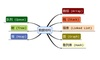数据结构 订阅